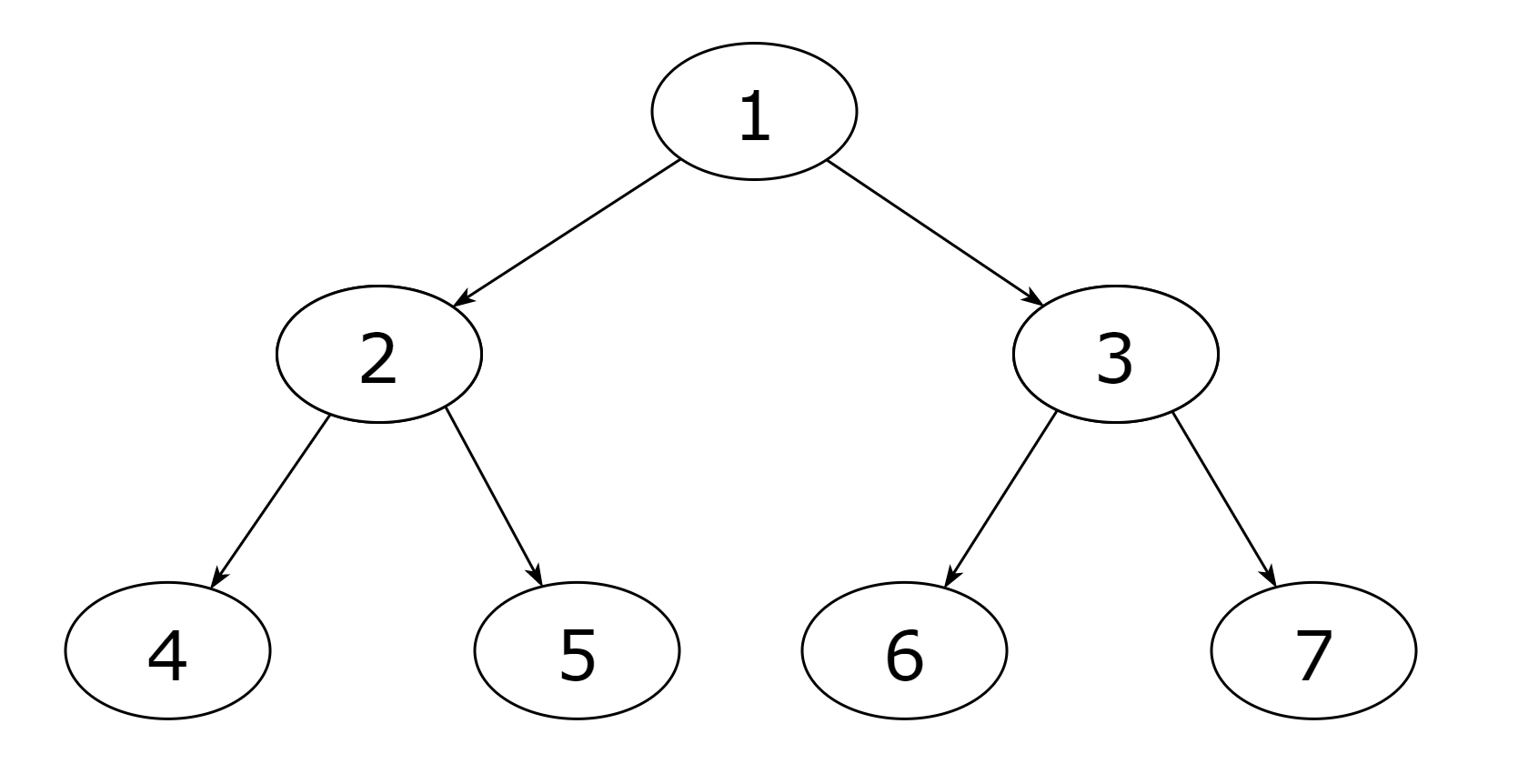New update is available. Click here to update.

# Create a binary tree from postorder and preorder traversal

Last Updated: 24 Mar, 2021
Difficulty: Moderate

## PROBLEM STATEMENT

#### You are given the ‘POSTORDER’ and ‘PREORDER’ traversals of a binary tree. The binary tree consists of ‘N’ nodes where each node represents a distinct positive integer named from ‘1’ to ‘N’. The task is to return the root node of any binary tree that matches the given ‘POSTORDER’ and ‘PREORDER’ traversals.

##### Example:
``````‘POSTORDER’ = [4, 5, 2, 6, 7, 3, 1]
‘PREORDER’ = [1, 2, 4, 5, 3, 6, 7]
A binary tree that matches the given ‘POSTORDER’ and ‘PREORDER’ traversal is:
````````````So, create this binary tree and return the root node ‘1’.
``````
##### Note:
``````1. You can return any binary tree that matches the given ‘POSTORDER’ and ‘PREORDER’ traversals.

2. You can always construct a valid binary tree from the ‘POSTORDER’ and ‘PREORDER’ traversals.
``````
##### Input format:
``````The first line of input contains an integer ‘T’ which denotes the number of test cases. Then, the ‘T’ test cases follow.

The first line of each test case contains an integer ‘N’ denoting the number of nodes in the binary tree.

The second line of each test case contains ‘N’ space-separated integers denoting the ‘POSTORDER’ traversal of the binary tree.

The third line of each test case contains ‘N’ space-separated integers denoting the ‘PREORDER’ traversal of the binary tree.
``````
##### Output format:
``````For every test case, return the root node of the newly created binary tree. The printed output will be the ‘POSTORDER’ and ‘PREORDER’ traversals of the returned tree, each printed on a new line.
``````
###### Note:
``````You do not need to print anything; it has already been taken care of. Just implement the function.
``````
##### Constraints:
``````1 <= T <= 10
1 <= N <= 10^3

Time limit: 1 second
``````## Approach 1

We can create a unique binary tree from (‘INORDER’ and ‘PREORDER’) traversals or
(‘INORDER’ and ‘POSTORDER’) traversals. But, (‘POSTORDER’ and ‘PREORDER’) traversals don’t give us enough information to create a unique binary tree. For example, the following two trees produce the same ‘POSTORDER’ and ‘PREORDER’ traversal sequence:

‘PREORDER’ = [1, 2, 3, 4]

‘POSTORDER’ = [4, 3, 2, 1]

So, we create the binary tree assuming that ‘PREORDER[i+1]’ is always the left child of ‘PREORDER[i]’.

[ ROOT NODE ] [ LEFT SUBTREE ...] [ RIGHT SUBTREE ...] => PREORDER

[ LEFT SUBTREE ...] [ RIGHT SUBTREE ...] [ ROOT NODE ] => POSTORDER

We know that the root of the tree is the first element in ‘PREORDER’ traversal, i.e., ‘PREORDER’ and the last element in ‘POSTORDER’ traversal,i.e., ‘POSTORDER[n-1]’. Then, the next element in ‘preorder’, i.e., ‘preorder’, is the left child of the root node. So, all nodes before index ‘i’ in ‘POSTORDER’ (where ‘POSTORDER[i]’ = ‘preorder’) must be present in the left subtree of the root node. All nodes from index ‘i+1’ to index ‘n-3’ must be present in the right subtree (because ‘POSTORDER[n-2]’ is the right child of the root node). Now, we recursively build the left and right subtree and link them to the root node. Consider the following example:

‘PREORDER’ = [1, 2, 4, 5, 3, 6, 7, 8]

‘POSTORDER’ = [4, 5, 2, 7, 8, 6, 3, 1]

From ‘PREORDER’, we get that ‘2’ is the left child of the root node. The left subtree size is three as there are two elements before ‘2’ in ‘POSTORDER’.

Left child of ROOT: ‘2’, and left subtree:

‘PREORDER’ = [2, 4, 5]

‘POSTORDER’ = [4, 5, 2]

In ‘POSTORDER’, the nodes after ‘2’ up to the second last element belong to the right subtree. The second last element in ‘POSTORDER’ is the right child of the root node. Therefore, there are four nodes in the right subtree.

Right child of ROOT: ‘3’, and right subtree:

‘PREORDER’ = [3, 6, 7, 8]

‘POSTORDER’ = [7, 8, 6, 3]

Link the root node to its left and right child. Similarly, solve for the left subtree and the right subtree recursively. Write a recursive helper function ‘BUILD_TREE’ which takes three parameters starting index of ‘POSTORDER’ (i.e., ‘POST_INDEX’), starting index of ‘PREORDER’ (i.e., ‘ST’), and the last index of ‘PREORDER’ (i.e., ‘END’).

• ‘ROOT = BUILD_TREE(0, 0, n - 1)’. The ‘BUILD_TREE’ function recursively creates a binary tree based on ‘PREORDER’ and ‘POSTORDER’, and returns its ‘ROOT’ node.
• Return ‘ROOT’ as the answer.

The ‘BUILD_TREE(integer POST_INDEX, integer ST, integer END)’ function:

• If ‘ST’ is greater than ‘END’, then end the recursion:
• Return ‘NULL’.
• Create a new node named ‘ROOT’ with the value ‘PREORDER[ST]’. This is the root node of the subtree from ‘PREORDER[ST]’ to ‘PREORDER[END]’.
• If ‘ST +1’ is greater than ‘END’, then this subtree has no child nodes:
• Return ‘ROOT’.
• Initialize an integer ‘INDEX = POST_INDEX’. Use this to find the position of ‘PREORDER[ST+1]’ (i.e., the left child of ‘ROOT’) in ‘POSTORDER’.
• Run a loop until ‘PREORDER[ST+1]’ is not equal to ‘POSTORDER[INDEX]’:
• ‘INDEX += 1’
• Initialize an integer ‘SIZE = INDEX - POST_INDEX + 1’. This is the size of the left subtree.
• ‘(ROOT→LEFT) = BUILD_TREE(POST_INDEX, ST + 1, ST + SIZE)’. Recursively create the left subtree and link it to the ‘ROOT’ node.
• ‘(ROOT→RIGHT) = BUILD_TREE(INDEX + 1, ST + SIZE + 1, END)’. Recursively create the right subtree and link it to the ‘ROOT’ node.
• Return ‘ROOT’ node.